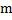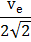# A solid sphere of uniform density and radiusapplies a gravitational force of attraction equal toon a particle placed at, distancefrom the centreof the sphere. A spherical cavity of radiusis now made in the sphere as shown in figure. The sphere with cavity now applied an gravitational forceon same particle placed at. The ratiowill bea) 1/2 b) 7/ 9 c) 3 d) 7

## Question ID - 55345 :- A solid sphere of uniform density and radiusapplies a gravitational force of attraction equal toon a particle placed at, distancefrom the centreof the sphere. A spherical cavity of radiusis now made in the sphere as shown in figure. The sphere with cavity now applied an gravitational forceon same particle placed at. The ratiowill bea) 1/2 b) 7/ 9 c) 3 d) 7

3537

(b)

Gravitational force due to solid sphere,whereandare mass of the solid sphere and particle respectively andis the radius of the sphere. The gravitational force on particle due to sphere with cavity = force due to solid sphere creating cavity, assumed to be present above at that positionSo,Next Question :
 The escape velocity of a body from earth’s surface is. The escape velocity of the same body from a height equal tofrom earth’s surface will be a)b)c)d)